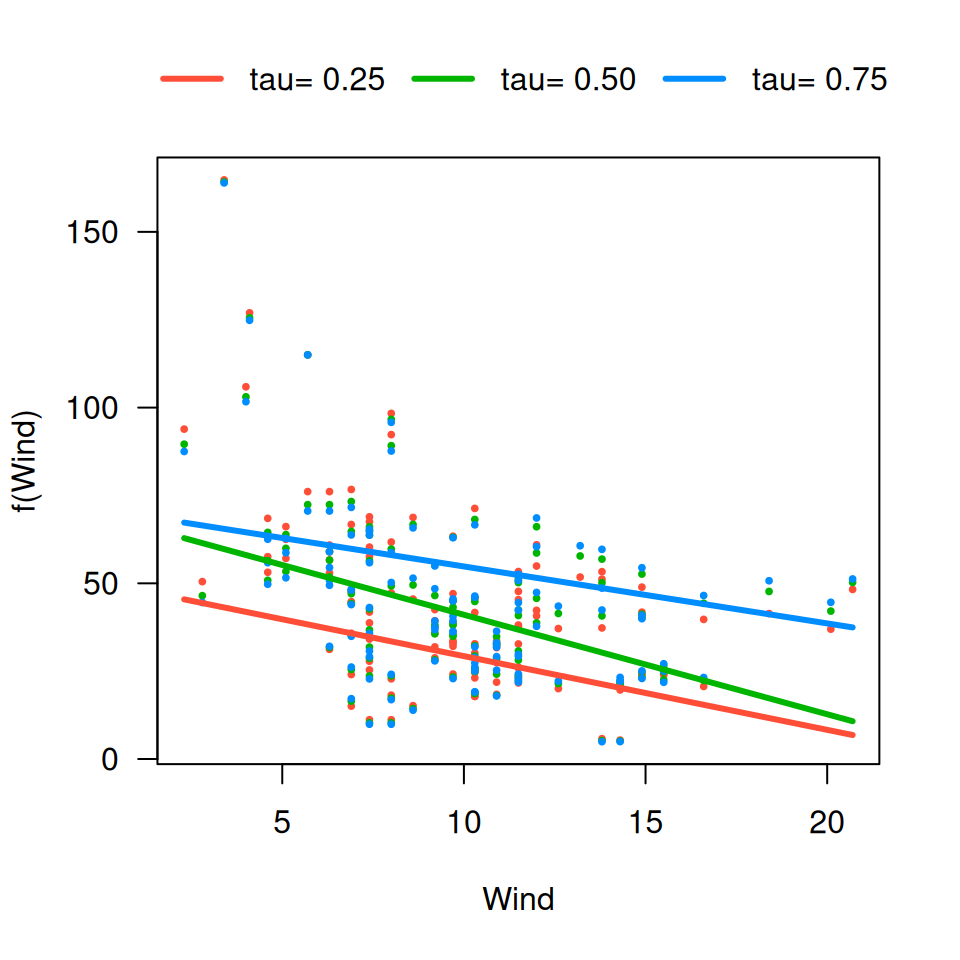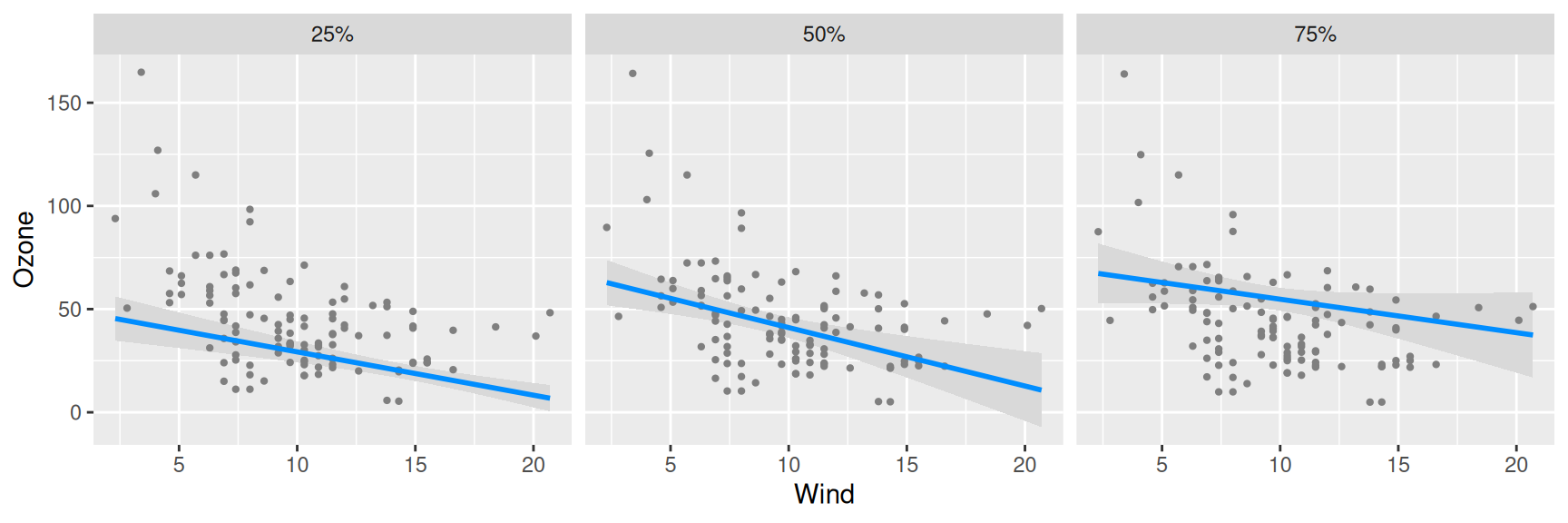Multiple visreg objects can be bundled together in an object of class visregList; for example, when you submit visreg(fit), you get a visregList, one visreg object for each predictor in the model. visregLists can also be used for handling models with multiple outcomes.

For example, suppose we fit a multinomial regression model using the nnet package:

library(nnet)
airquality$Heat <- cut(airquality$Temp,3,labels=c("Cool","Mild","Hot"))
fit <- multinom(Heat ~ Wind + Ozone, airquality)

By default, visreg(fit, "Ozone") would create three separate plots, one for each level of the outcome, Heat. By specifying collapse=TRUE, we collapse the list down to a single visreg object which can be plotted using the methods described here. For example:

visreg(fit, "Ozone", collapse=TRUE, overlay=TRUE, ylab="Probability",
ylim=c(0,1), partial=FALSE, rug=2)Another example is quantile regression using the quantreg package. Here, there is only one outcome, but we could be interested in modeling several different quantiles. The collapse option is used similarly here:

library(quantreg)
fit <- rq(Ozone ~ Wind + Temp, tau=c(.25, .5, .75), data=airquality)
v <- visreg(fit, "Wind", overlay=TRUE, collapse=TRUE)NOTE: quantreg does not return standand errors if you specify multiple quantiles of interest. To obtain them, you must construct the visregList manually:

fit1 <- rq(Ozone ~ Wind + Temp, tau=.25, data=airquality)
fit2 <- rq(Ozone ~ Wind + Temp, tau=.5, data=airquality)
fit3 <- rq(Ozone ~ Wind + Temp, tau=.75, data=airquality)
v <- visregList(visreg(fit1, "Wind", plot=FALSE),
visreg(fit2, "Wind", plot=FALSE),
visreg(fit3, "Wind", plot=FALSE),
labels=c("25%", "50%", "75%"), collapse=TRUE)
plot(v, ylab="Ozone", gg=TRUE)
# Loading required namespace: ggplot2Notice the use of the labels argument to label the elements of the list.